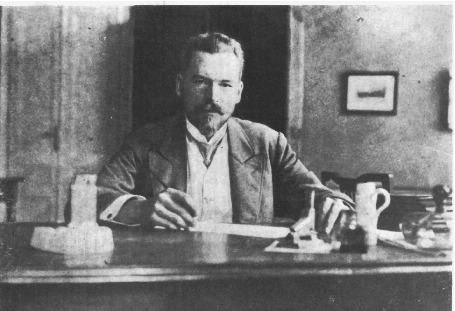#31st Marian Smoluchowski Symposium on Statistical Physics

2-7 September 2018
Europe/Warsaw timezone

## When boundary conditions at a thin membrane create nonmarkovian normal diffusion

3 Sep 2018, 17:15
20m
talk

### Speaker

Dr. Tadeusz Kosztolowicz (Inititute of Physics, Jan Kochanowski University, Świętokrzyska 15, 25-406 Kielce, Poland)

### Description

In the paper  one of the boundary conditions at a thin membrane for normal diffusion has been obtained directly from experimental data. This condition contains the Riemann--Liouville fractional derivative of the $1/2$ order and reads $P(0^-,t|x_0,0)=\left(a+b\frac{\partial^{1/2}}{\partial t^{1/2}}\right)P(0^+,t|x_0,0)$, the membrane is located at $x=0$, the fundamental solution to normal diffusion equation $P(x,t|x_0,0)$ is interpreted as a probability density of finding a diffusing particle at the point $x$ at time $t$ under condition that in the initial moment $t=0$ the particle was in the initial position $x_0$. The fractional derivative causes that particle's transition through the membrane can be interpreted as a `long memory' process. The question arises: does the presence of the fractional derivative in a boundary condition cause that the diffusion process in the membrane system is nonmarkovian? We show that the appearance of an obstacle such as a thin partially permeable membrane can change the nature of normal diffusion which would be considered as a nonmarkovian process. If the process is markovian, the fundamental solution fulfills the Bachelier-Smoluchowski-Chapman-Kolmogorov (BSCK) equation $P(x,t|x_0,0)=\int_{-\infty}^\infty dx' P(x,t|x',t')P(x',t'|x_0,0)$. We conduct the consideration in terms of the Laplace transform $\hat{f}(s)=\int_0^\infty {\rm e}^{-st}f(t)dt$. Boundary conditions at an asymmetric membrane depend on in which part of the system the initial position of the particle is placed. We assume the boundary conditions at the membrane in terms of the Laplace transform as follows $\hat{P}(0^+,s|x_0, 0)=\hat{\Phi_1}(s) \hat{P}(0^-,s|x_0,0)$, $\hat{J}(0^+,s|x_0,0)=\hat{\Xi_1}(s)\hat{J}(0^-,s|x_0,0)$, for $x_0<0$ and $\hat{P}(0^-,s|x_0,0)\hat{\Phi_2}(s)\hat{P}(0^+,s|x_0,0)$, $\hat{J}(0^-,s|x_0,0)=\hat{\Xi_2}(s)\hat{J}(0^+,s|x_0,0)$, for $x_0>0$, where $\hat{J}(x,s|x_0,0)=-D\partial \hat{P}(x,s|x_0,0) / \partial x$ is the Laplace transform of diffusive flux. The above equations can be considered as general form of boundary conditions at a thin membrane . We will derive the equations that must be fulfilled by the functions $\Xi_{1,2}(s)$ and $\Phi_{1,2}(s)$ so that the function $\hat{P}(x,s|x_0,0)$ satisfies the BSCK equation. The functions $\Xi_{1,2}(s)$ and $\Phi_{1,2}(s)$ are measurable experimentally by means of the method presented in . Thus, it can be checked experimentally if the boundary conditions violate Markov property for the normal diffusion process. The measure of nonmarkovianity is the normalized autocorrelation function . We will also discuss the relation between boundary conditions at the membrane and the measure of nonmarkovianity.  T. Kosztołowicz, S. Wąsik, K.D. Lewandowska, Phys. Rev. E 96, 010101(R) (2017).  A. Fuliński, EPL 118, 60002 (2017); J. Phys. A 50, 054002 (2017).

### Primary author

Dr. Tadeusz Kosztolowicz (Inititute of Physics, Jan Kochanowski University, Świętokrzyska 15, 25-406 Kielce, Poland)

### Co-author

Dr. Katarzyna D. Lewandowska (Department of Radiological Informatics and Statistics, Medical University of Gdańsk, Tuwima 15, 80-210 Gdańsk, Poland)

### Presentation Materials

There are no materials yet.
###### Your browser is out of date!

Update your browser to view this website correctly. Update my browser now

×